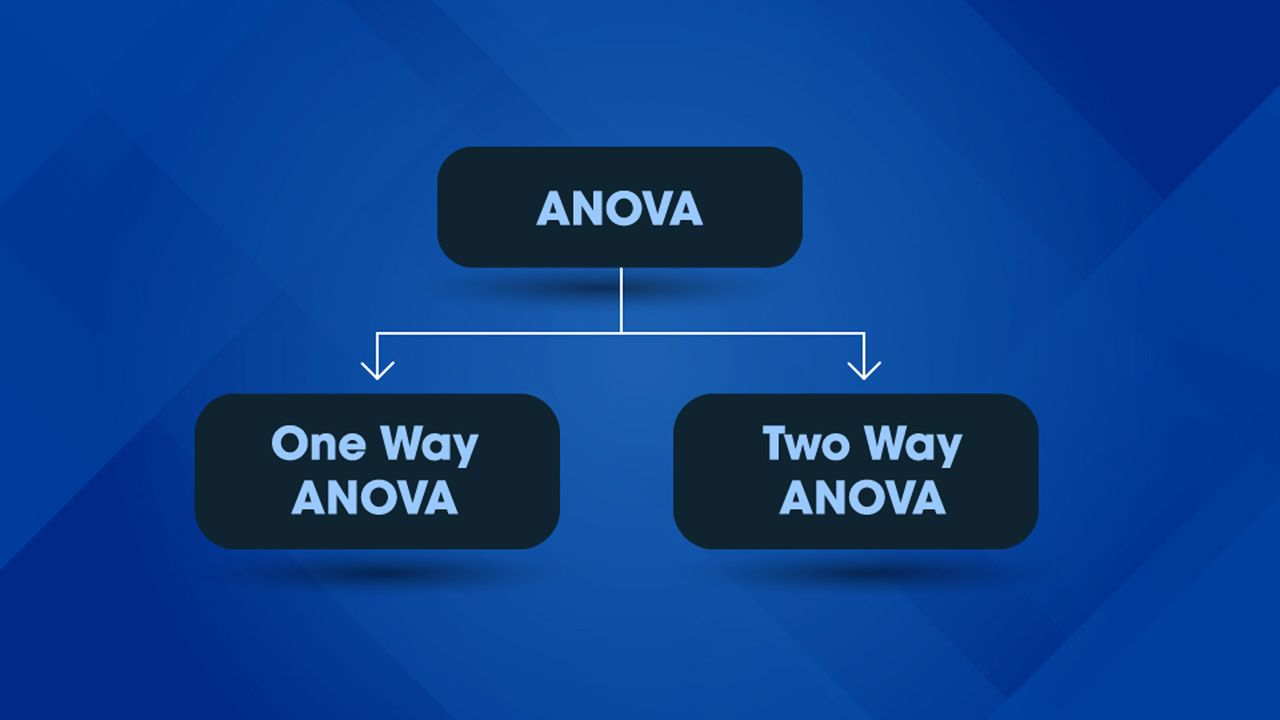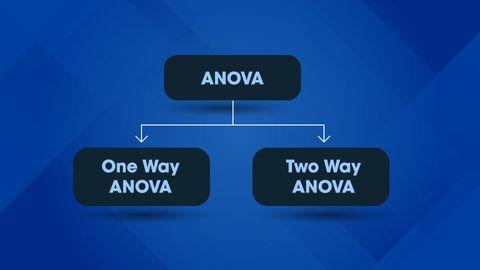We use cookies to provide you with a better experience. You can read our Cookie Policy here.Article

# One-Way vs Two-Way ANOVA: Differences, Assumptions and HypothesesArticle

# One-Way vs Two-Way ANOVA: Differences, Assumptions and Hypotheses

Complete the form below and we will email you a PDF version of "One-Way vs Two-Way ANOVA: Differences, Assumptions and Hypotheses"

First Name*
Last Name*
Country*
Company Type*
Job Function*
Would you like to receive further email communication from Technology Networks?

Technology Networks Ltd. needs the contact information you provide to us to contact you about our products and services. You may unsubscribe from these communications at any time. For information on how to unsubscribe, as well as our privacy practices and commitment to protecting your privacy, check out our Privacy Policy

A key statistical test in research fields including biology, economics and psychology, analysis of variance (ANOVA) is very useful for analyzing datasets. It allows comparisons to be made between three or more groups of data. Here, we summarize the key differences between these two tests, including the assumptions and hypotheses that must be made about each type of test.

There are two types of ANOVA that are commonly used, the one-way ANOVA and the two-way ANOVA. This article will explore this important statistical test and the difference between these two types of ANOVA.

## What is a one-way ANOVA?A one-way ANOVA is a type of statistical test that compares the variance in the group means within a sample whilst considering only one independent variable or factor. It is a hypothesis-based test, meaning that it aims to evaluate multiple mutually exclusive theories about our data. Before we can generate a hypothesis, we need to have a question about our data that we want an answer to. For example, adventurous researchers studying a population of walruses might ask “Do our walruses weigh more in early or late mating season?” Here, the independent variable or factor (the two terms mean the same thing) is “month of mating season”. In an ANOVA, our independent variables are organised in categorical groups. For example, if the researchers looked at walrus weight in December, January, February and March, there would be four months analyzed, and therefore four groups to the analysis.

A one-way ANOVA compares three or more than three categorical groups to establish whether there is a difference between them. Within each group there should be three or more observations (here, this means walruses), and the means of the samples are compared.

## What are the hypotheses of a one-way ANOVA?

In a one-way ANOVA there are two possible hypotheses.

• The null hypothesis (H0) is that there is no difference between the groups and equality between means (walruses weigh the same in different months).
• The alternative hypothesis (H1) is that there is a difference between the means and groups (walruses have different weights in different months) .

## What are the assumptions and limitations of a one-way ANOVA?

• Normality – that each sample is taken from a normally distributed population
• Sample independence – that each sample has been drawn independently of the other samples
• Variance equality – that the variance of data in the different groups should be the same
• Your dependent variable – here, “weight”, should be continuous – that is, measured on a scale which can be subdivided using increments (i.e. grams, milligrams)

## What is a two-way ANOVA?A two-way ANOVA is, like a one-way ANOVA, a hypothesis-based test. However, in the two-way ANOVA each sample is defined in two ways, and resultingly put into two categorical groups. Thinking again of our walruses, researchers might use a two-way ANOVA if their question is: “Are walruses heavier in early or late mating season and does that depend on the sex of the walrus?” In this example, both “month in mating season” and “sex of walrus” are factors – meaning in total, there are two factors.  Once again, each factor’s number of groups must be considered – for “sex” there will only two groups “male” and “female”.

The two-way ANOVA therefore examines the effect of two factors (month and sex) on a dependent variable – in this case weight, and also examines whether the two factors affect each other to influence the continuous variable.

## What are the assumptions and limitations of a two-way ANOVA?

• Your dependent variable – here, “weight”, should be continuous – that is, measured on a scale which can be subdivided using increments (i.e. grams, milligrams)
• Your two independent variables – here, “month” and “sex”, should be in categorical, independent groups.
• Sample independence – that each sample has been drawn independently of the other samples
• Variance Equality – That the variance of data in the different groups should be the same
• Normality – That each sample is taken from a normally distributed population

## What are the hypotheses of a two-way ANOVA?

Because the two-way ANOVA consider the effect of two categorical factors, and the effect of the categorical factors on each other, there are three pairs of null or alternative hypotheses for the two-way ANOVA. Here, we present them for our walrus experiment, where month of mating season and sexare the two independent variables.

• H0: The means of all month groups are equal
• H1: The mean of at least one month group is different

• H0: The means of the sex groups are equal
• H1: The means of the sex groups are different

• H0: There is no interaction between the month and gender
• H1: There is interaction between the month and gender

## Interactions in two-way ANOVA

These last two hypotheses, of there being (or not being) interactions in a two-way ANOVA, refer to how the two variables in the study affect each other.

This is most easily explained by going back to our walruses.

If the researchers found that male walrus weight significantly decreased between December and March, but female walrus weight remained steady or slightly increased, subsequent statistical analysis may conclude that there was an interaction between the two independent variables of month and sex.

These effects are not to be ignored. If we put the interactions to one side, with the results mentioned above, an incomplete analysis might conclude that walruses in general lost weight over mating season, which would ignore a reality that the decrease was driven by changes to male walrus weight. Another example could be the efficacy of a candidate drug for a disease; you can see how proper modeling of interaction effects can become critical to many biological research studies.

## Summary: differences between one-way and two-way ANOVA

The key differences between one-way and two-way ANOVA are summarized clearly below.

1. A one-way ANOVA is primarily designed to enable the equality testing between three or more means. A two-way ANOVA is designed to assess the interrelationship of two independent variables on a dependent variable.

2. A one-way ANOVA only involves one factor or independent variable, whereas there are two independent variables in a two-way ANOVA.

3. In a one-way ANOVA, the one factor or independent variable analyzed has three or more categorical groups. A two-way ANOVA instead compares multiple groups of two factors.

4. One-way ANOVA need to satisfy only two principles of design of experiments, i.e. replication and randomization. As opposed to two-way ANOVA, which meets all three principles of design of experiments which are replication, randomization and local control.

## One-Way ANOVATwo-Way ANOVADefinitionA test that allows one to make comparisons between the means of three or more groups of data.A test that allows one to make comparisons between the means of three or more groups of data, where two independent variables are considered. Number of Independent VariablesOne.Two. What is Being Compared?The means of three or more groups of an independent variable on a dependent variable.The effect of multiple groups of two independent variables on a dependent variable and on each other. Number of Groups of Samples Three or more.Each variable should have multiple samples.

Meet the AuthorRuairi J Mackenzie
Senior Science Writer